# Quiz 19: Managing Net Working Capital

Business

With the information given, currently each of the four affiliates is holding cash equal to the mean of their perceived demand plus three standard deviations. The total demand for cash by each affiliate as followings:The total demand for cash sums these demands to give €238,500,000. If we summarize the cash management, the sum of the means and variance would be sum of the variance because cash are independent for each other. Thus, the cash of centralized cash pool as followings: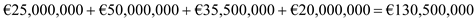The variance of the demand for cash of centralized cash pool is as followings: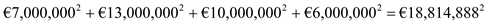Thus, total demand for cash by the centralized pool is as follows: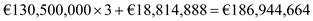The demand for cash from the centralized cash pool is as follows: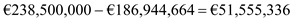Therefore, total demand for cash in centralized cash pool is less than the total demand for cash from the separate affiliates.

The difference amount between a firm's current assets and current liabilities at a point in time is generally known as net working capital. The current assets consists collection of cash, marketable securities, accounts receivable, inventories, prepaid expenses, accrued income etc. And current liabilities consists short term debt, and account payable, unearned income, outstanding expenses etc. It is calculated by using following formula: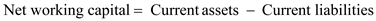Net working capital or the difference of current assets and current liability got financed from long-term sources of finance, therefore is a type of investment. This investment a firm use to produce revenue in the future. So, it is an important investment for a firm.

a. With the information given, we can drive the minimum transfer from the cumulative monthly payments as followings: If we add the total payments for each affiliate, to find the payments as following:With no netting system, total payment would be the sum of individual payment of affiliates €161 million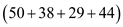. Add the total receipts for each affiliate, to find total receipts as following:With no netting system, total receipts would be the sum of individual receipts of affiliates €161 million. Now, subtract each affiliates total receipts from its total payments through a multilateral netting system as follows:Therefore, total of €19(2+17) million must be transferred among the affiliates. This can be achieved by having the Swedish affiliate pay €2 Million to Italian affiliate and the Spanish affiliate pay €9 million to the Italian affiliate and €8 million to the French affiliate. b. With the information given, calculate the save amount by company as followings: Now, calculate the difference in the total amount transferred without and with a multilateral netting system as follows: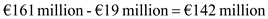Now, if the transaction cost is 0.45% of the amount transferred, the total saving is as following: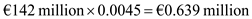Therefore, total saving is €0.639million.